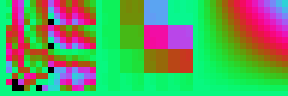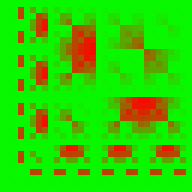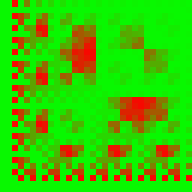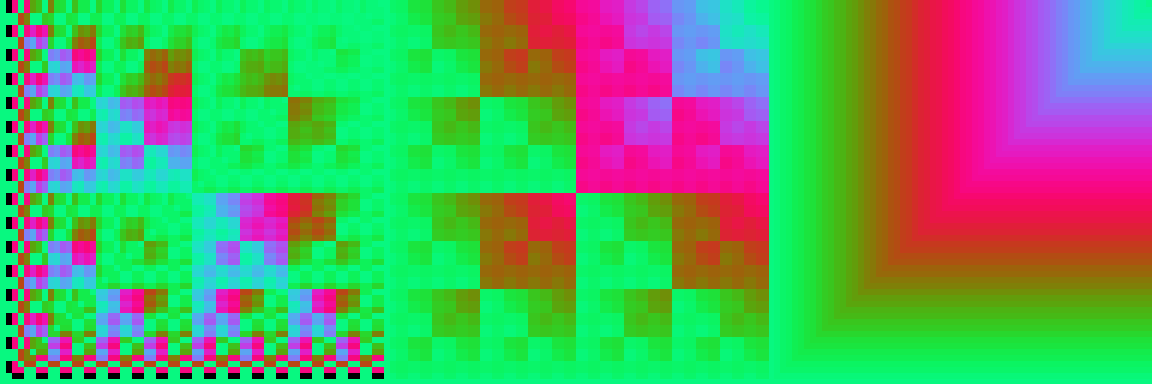Portfolio Noah Vawter: Using Odd Math to your advantage

by Noah Vawter

## Implementation of a 2-pole State Variable Filter with Inaccurate Math Functions to Lower CPU Requirements and Generate Artifacts

### Logic Reduction

Imagine for a moment two N-bit numbers: X[(N-1)<->0] and Y[ (N-1)<->0].

If you were to write the logic equations for each bit of the their product Z=X*Y, you'd get this:

• z = x & y
• z = (y & x) xor (y) & x)
• ..
• z[(N-1)] = ...

Observe that the LSB terms are rather simple, while the MSB terms, since they're the result of much carrying, are more complex. The same observation holds true for many functions of two variables, such as atan2, sqrt(x*x+y*y), etc.

### Odd Math

Odd Math is all about replacing those complex, correct logic functions with simplified versions that still give interesting results.

Why? Don't you want accurate math functions? Not always. In fact, in the physical world it's impossible to have perfectly accurate math. Odd Math lets you exchange some accuracy for MASSIVE reductions in cpu time.

I use genetic algorithms to grow the logic equations. They are generally able to execute in a single clock cycle with no pipelining. The accuracy can be surprising. I've gotten as close as 28.5% accurate with a 6-bit multiply so far! The examples below were done with a 44% accurate 7-bit multiplier.

## What This Looks Like Graphically

In these graphs, the rightmost section is the target function, the middle section is the generated function, and the left section is the percent error. Black indicates the error is >100%. The target function is is X multiplied by Y. Note: this is normally a symmetric function, but the genetic algorithm found it could gain accuracy by introducing slight assymmetry.

Initial (bitwise) estimate of the function:First generation of genetic algorithm. Notice how much less black area exists in the error graph:3rd generation. There is now even less black area, and the error is tightened up:4th generation. No visible changes in this graph, but the error has been reduced even more:## Can I actually hear the effects on audio?

The first half of this project was a 700-line perl program that generated the function approximations. The second half of this project was an implementation the familiar 2-pole svf filter to use my "badmultiply" in place of its normal multiplies. The addition functions were kept pure. A control was added for degree of badness. You can hear the results of various settings on sinusoidal input here:

The samples below are the output of a 2-pole SVF filter sweep on an input signal composed of 4 sinusoids. The sinusoids form a root, fifth, octave, fifth chord. I detune the pitches ever so slightly for a good reason. The chorusing forces the otherwise harmonic waveforms to vary their relative phases, allowing me to more carefully examine the non-linear effects the approximations.

• With 0% oddmath mixed in nice and squeaky clean.
• With 50% oddmath mixed in notice the small amount of fliter cutoff frequency-related noise
• With 75% odddmath mixed in the burp at the beginning is shifted to the mid-range. Also chaos at the very end.
• With 95% oddmath mixed in just a mess, but still related to Fcutoff.
• With 99% oddmath mixed in Ummmmmmmmmmmmmmmmm....

BTW, the burp at the beginning is the filter resonating at 0.05Fs. I decided to leave it in, because it actually goes away when the parameters change.

## Beyond: One-Dimensional Functions

This same algorithm can be used to generate one dimensional functions. I have generated resonably accurate sine waves with very simple, quickly-executing logic equations.

## Some Other Neat-Looking GraphsThis is an approximation for addition. I think the fractal structure is very beautiful.Click Me!